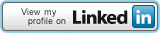## Connect Me## Pages

### SLC QBASIC- Array problems

WAP to ask user 20 different integers and print the sum of positive numbers only.

DECLARE SUB SUM(N())
DIM N(20)
CLS
PRINT "Enter 20 integers"
FOR I= 1 TO 20
INPUT "Number";N(I)
NEXT I
CALL SUM(N())
END

SUB SUM(N())
FOR J=1 TO 20
IF N(J)>0 THEN S=S+N(J)
NEXT J
PRINT "Sum of positive numbers is ";S
END SUB

WAP to ask user 10 different numbers and print the greatest number.

DECLARE SUB GREAT(N())
DIM N(10)
CLS
PRINT "Enter 10 numbers"
FOR I= 1 TO 10
INPUT "Number";N(I)
NEXT I
CALL GREAT(N())
END

SUB GREAT(N())
FOR J=1 TO 10
IF N(J)>G THEN G=N(J)
NEXT J
PRINT "Greastest number is ";G
END SUB
Ą